# SSC Reasoning Ability Practice Questions (Day-67)

Dear Aspirants, The SSC Exams are going on at national level. Millions of candidates have eagerly prepared for the ongoing SSC exams such as CGL, CHSL, MTS, CPO, JE etc. SSC exams are usually conducted by the Staff Selection Commission to recruit the eligible candidates for various Central government departments. Mostly the selection process for all the SSC exams consists of the single or double phase of written examination based on the examination. Due to that, the written exam consisted of a certain syllabus and exam pattern. By following those regulations, the written exam has been conducted through an online mode. Based on the latest updated exam pattern and syllabus, here we have drafted the Reasoning Ability questions on a daily basis. So aspirants start your practice session with us and mould your skill in a perfect shape.

Start Quiz

Direction (1): In the following question, select the number which can be placed at the sign of question mark (?) from the given alternatives.

1)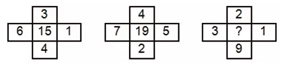(a) 16

(b) 17

(c) 18

(d) 19

Direction (2-3): How many triangles are there in the given figure?

2)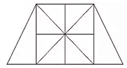(a) 16

(b) 18

(c) 20

(d) 24

3)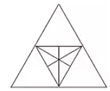(a) 16

(b) 18

(c) 19

(d) 20

Direction (4-5): In each of the following question below are given some statements followed by some conclusions. Taking the given statements to be true even if theyseem to be at variance from commonly known facts, read all the conclusions and then decide which of the given conclusion logically follows the given statements.

4) Statements:    I) All cups are plates.

II) No plate is spoon.

III) Some spoons are pens.

Conclusions: I) Some pens are cups.

II) Some cups are spoons.

III) No cup is spoon.

IV) Some pens are not cups.

(a) Only conclusion (II) and (III) follow

(b) Only conclusion (III) and (IV) follow

(c) Only conclusion (I) and (IV) follow

(d) Only conclusion (I), (II) and (III) follow

5) Statements:    I)
All pens are pencils.

II) No pencil is eraser.

III) Some cups are erasers.

Conclusions: I) Some cups are not pencils.

II) Some cups are not pens.

III) Some pencils are not cups.

IV) No pen is eraser.

(a) Only conclusion (I), (II) and (III) follow

(b) Only conclusion (II) and (IV) follow

(c) Only conclusion (I), (II) and (IV) follow

(d) All conclusions follow

Direction (6-7): From the given options, which answer figure can be formed by folding the figure given in the question ?

6)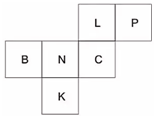(a)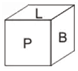(b)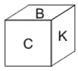(c)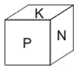(d)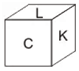7)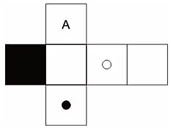(a)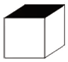(b)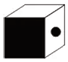(c)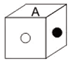(d)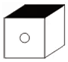8) In the given, which number represents poisonous insects which are not black?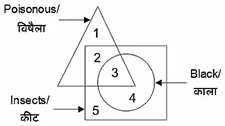(a) 2

(b) 3

(c) 4

(d) 5

9) In the given figure, which alphabet/alphabets represent old cars?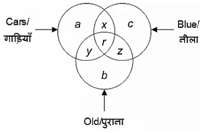(a) y and r

(b) y

(c) a, y and b

(d) a and b

10) In the given figure, how many metallic balls are also heavy?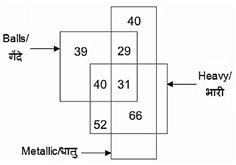(a) 97

(b) 71

(c) 31

(d) 66

(6 + 3 + 1 + 4) + 1 = 15

(7 + 4 + 5 + 2) + 1 = 19

(3 + 2 + 1 + 9) + 1 = 16

20 triangles

20 triangles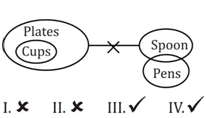Only conclusion III and IV follow.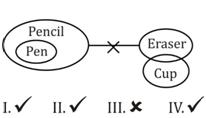Only conclusion I, II and IV follows.

Opposite faces are—

B ↔ C

K ↔ L

N ↔ P

Option (a) can be formed.

Opposite faces are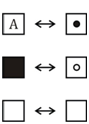Option (b) can be formed.

2 represents poisonous insects which are not black.

y and r represents old cars.